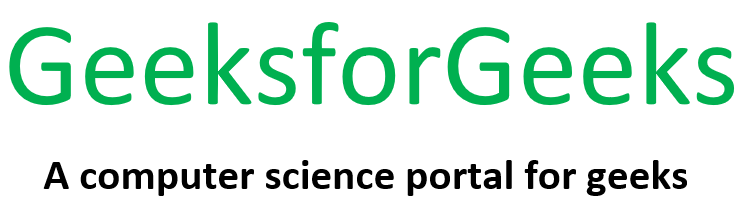# PHP | Imagick gaussianBlurImage() Function

• Last Updated : 09 Jul, 2019

The Imagick::gaussianBlurImage() function is an inbuilt function in PHP which is used to blur an image. This function convolves the image with a Gaussian operator of the given radius and standard deviation (sigma).

Note: For reasonable results, the radius should be larger than sigma.

Syntax:

`bool Imagick::gaussianBlurImage( \$radius, \$sigma, \$channel = Imagick::CHANNEL_DEFAULT )`

Parameters: This function accepts three parameters as mentioned above and described below:

• \$radius (float type): This parameter gives the radius of the Gaussian area in pixels, excluding the center pixel.
• \$sigma (Float type): This parameter gives the standard deviation of the Gaussian area in pixels.
• \$channel (Int type): This parameter gives a channel (that is valid) as per our requirement. To apply more than one channel, use bitwise operator to combine channel-type constants.

Return Value: This function returns True on success.

Errors: This function throws ImagickException on error.

Original Image:Now, we will write a PHP program that illustrates the Imagick::gaussianBlurImage() function in PHP to blur the above image.

Program: This program uses Imagick::gaussianBlurImage() function to blur the image.

 `gaussianBlurImage(10, 8, Imagick::CHANNEL_DEFAULT); ` `      `  `// Image header ` `header(``"Content-Type: image/jpg"``); ` `      `  `// Display the output image ` `echo` `\$imagick``->getImageBlob(); ` `  `  `?> `

Output:My Personal Notes arrow_drop_up
Recommended Articles
Page :